# 狩龙战纪属性点爆伤收益与双刀小知识

《狩龙战纪》游戏中关于伤害的统计测试一直都有一些技术数据宅们在进行着，而这里将为大家测试关于属性点跟爆伤的收益，另外送上自己的双刀使用小知识。

《狩龙战纪》游戏中关于伤害的统计测试一直都有一些技术数据宅们在进行着，而这里将为大家测试关于属性点跟爆伤的收益，另外送上自己的双刀使用小知识。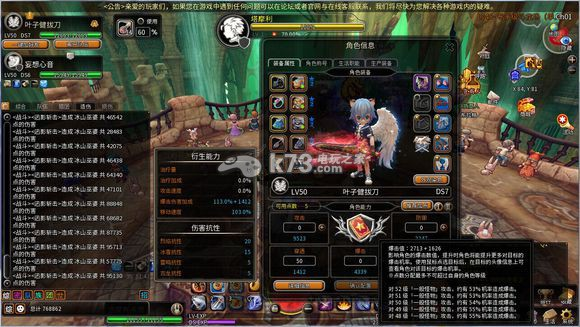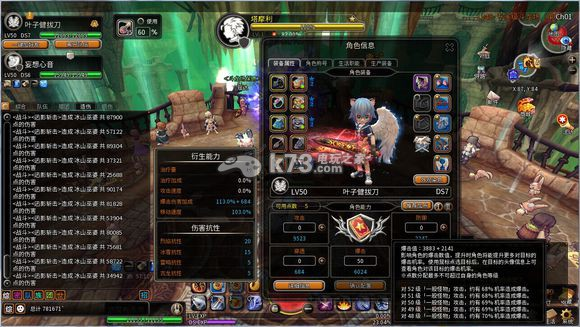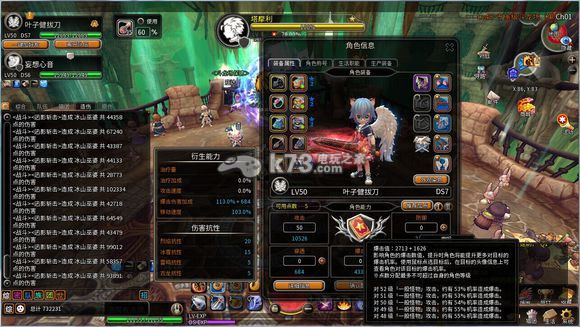(没暴击的最低+没暴击的最高)/2 去乘 100-暴击率 ----此为没暴击的总伤害

(暴击的最高+暴击的最低)/2 去乘 暴击率 ----此为应有暴击总伤害

【暴击加点】 暴击率 70%

(25688+58722)/2 * 30 = 1266150

(75681+90174)/2 * 70 = 5804925 总计:7071075

【穿透加点】 暴击率 55%

(28483+68682)/2 * 45 = 2186212.5

(87735+95713)/2 * 55 = 5044820 总计:7231032.5

【攻击加点】 暴击率 55%

(28773+67240)/2 * 45 = 2160292.5

(93891+102334)/2 * 55 = 5396187.5 总计:7556480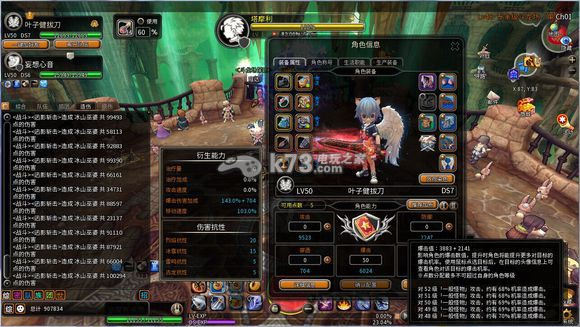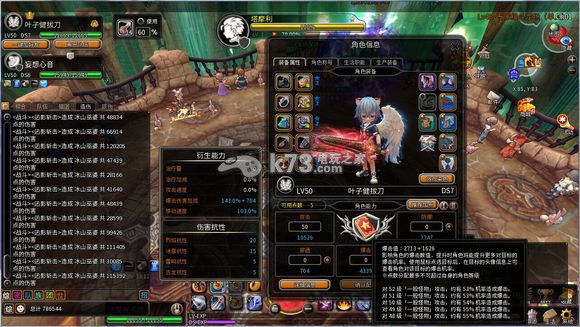【暴击加点】 暴击率 70%

(23137+66004)/2 * 30 = 1337115

(87921+100294)/2 * 70 = 6587525 总计:7924640

(75681+90174)/2 * 70 = 5804925 30%的爆伤偏差伤害=782600

【穿透加点】 暴击率 55%

(26686+71705)/2 * 45 = 2213797.5

(102180+117040)/2 * 55 = 6028550 总计:8242347.5

(87735+95713)/2 * 55 = 5044820 30%的爆伤偏差伤害=983730

【攻击加点】 暴击率 55%

(28166+66914)/2 * 45 = 2139300

(99426+120205)/2 * 55 = 6039852.5 总计:8179152.5

(93891+102334)/2 * 55 = 5396187.5 30%的爆伤偏差伤害=643665

【双刀小知识】

验证码：### 相关导航83k73手游